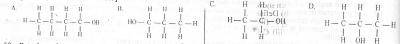# Chemistry 2017 Past Questions | WAEC

Study the following Chemistry past questions and answers for JAMBWAEC  NECO and Post-JAMB. Get prepared with official past questions and answers for upcoming examinations.

41. Calculate the mass of copper deposited if a current of 0.45A flows through CuSO4 solution for 1hour 15mins.   [Cu=64.0, S= 32.0, O =16.0, 1F= 96500C]

• A. 6.40g
• B. 0.67g
• C. 0.64g
• D. 0.45g

42. Classification of alkanols is based on the

• A. number of carbon atoms present in the compound
• B. molecular mass of the compound
• C. molecular formula of the compound
• D. number of alkyl groups bonded to the carbon having the hydroxyl group

43. The name of the compound $$C_{4}H_{9}COOC_{3}H_{7}$$ is

• A. propyl pentanoate
• B. pentyl propanoate
• C. butyl propanoate
• D. propyl butanoate

44. Which of the following pairs of gases are pollutants from car exhaust?

• A. Nitrogen(II) oxide and Carbon(IV) oxide
• B. Carbon(IV) oxide and Nitrogen
• C. Carbon(II) oxide and Sulphur(IV) oxide
• D. Carbon(II) oxide and oxygen

45. Fats and oils can be obtained from any of the following sources except

• A. paraffin oil
• B. animals
• C. groundnuts
• D. palm kernel

46. The condensation of two units of glucose will produce a dissacharide with the formula $$C_{12}H_{22}O_{11}$$ which is

• A. sucrose
• B. maltose
• C. galactose
• D. mannose

47. The IUPAC name for the compound $$ClCH_{2}CH_{2}OH$$ is

• A. 1- chloropropanol
• B. 2-chloropropanol
• C. 1-chloroethanol
• D. 2-chloroethanol
</li

48. The real harmful effect of the release of chlorofluorocarbons into the atmosphere is that it eventually causes

• A. excessive ultraviolet light from the sun to reach the earth surface
• B. excessive release of infrared light from the sun to the earth surface
• C. corrosive effect of the chemicals on humans and animals
• D. acidic effect of chemicals on humans

49.A primary alkanol has a molecular mass of 60. What is the structural formula of the compound? [C= 12.0, H= 1.0, O= 16.0)

50. Petroleum is a non-renewable source of energy because it

• A. is formed naturally
• B. is cheap
• C. can be recycled after use
• D. cannot be regenerated once used up

## Post suggestions Here

Subscribe
Notify ofInline FeedbacksFatima yushau
1 year ago

DwpDiscuz